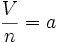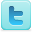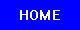Avogadro's Law
K-12 Experiments & Background Information
For Science Labs, Lesson Plans, Class Activities & Science Fair Projects
For Middle and High School Students & Teachers

 Avogadro's Law Experiments Avogadro's Law Concepts and Experiment - David N. Blauch, Davidson [View Experiment] Properties of Gases: Avogadro's Law - chemwiki [View Experiment] Verify Avogadro’s Law - Brigham Young University [View Experiment] The Molar Volume of Hydrogen Gas - East Carolina University [View Experiment] Avogadro's Law Experiment [View Experiment] The Ideal Gas Law: Measuring the Molar Volume of a Gas And The Universal Gas Constant [View Experiment] A Simple Demonstration for Ideal Gas Laws [View Experiment] Avogadro's Law Background Information Definition Avogadro's law is a gas law saying that "Equal volumes of ideal gases, at the same temperature and pressure, contain the same number of particles, or molecules." Thus, the number of molecules in a specific volume of gas is independent of the size or mass of the gas molecules. Topics of Interest Avogadro's law is one of the gas laws. The law is named after Amedeo Avogadro, who in 1811 hypothesized that equal volumes of gases, at the same temperature and pressure, contain the same number of particles, or molecules. Thus, the number of molecules in a specific volume of gas is independent of the size or mass of the gas molecules. The minor aspect of the law can be stated mathematically as:$\qquad {{V} \over {n}}= a$. -where: V is the volume of the gas. n is the number of moles in the gas. a is a constant. However, this above equation is just a trivial one, which is valid for all homogeneous substances, including homogeneous liquids and solids. This relation is easy to deduce, its validity was assumed before Avogadro's work. The most important consequence of Avogadro's law is the following: The ideal gas constant has the same value for all gases. This means that the constant const of$\frac{p_1\cdot V_1}{T_1\cdot n_1}=\frac{p_2\cdot V_2}{T_2 \cdot n_2} = const$ has the same value for all gases, independent of the size or mass of the gas molecules. This statement is nontrivial, and it containts Avogadro's ingenious insight in the nature of ideal gases. It took decades to prove Avogadro's law based on the kinetic theory of gases. One mole of an ideal gas occupies 22.4 liters (dm3) at STP (Standard conditions for temperature and pressure). This is often referred to as the molar volume of an ideal gas. Real gases may deviate from this value. The number of molecules in one mole is called Avogadro's number: approximately 6.022×1023 particles per mole. Avogadro's law, together with the combined gas law, forms the ideal gas law. The Ideal gas law is the equation of state of a hypothetical ideal gas. It is a good approximation to the behavior of many gases under many conditions, although it has several limitations. It was first stated by Émile Clapeyron in 1834 as a combination of Boyle's law and Charles's law. It can also be derived from kinetic theory, as was achieved (apparently independently) by August Krönig in 1856 and Rudolf Clausius in 1857. Source: Wikipedia (All text is available under the terms of the GNU Free Documentation License and Creative Commons Attribution-ShareAlike License.) Useful Links Science Fair Projects Resources Chemistry Science Fair Projects Books

Follow Us On:Privacy Policy - Site Map - About Us - Letters to the Editor

Comments and inquiries could be addressed to:
webmaster@julianTrubin.comLast updated: June 2013
Copyright © 2003-2013 Julian Rubin